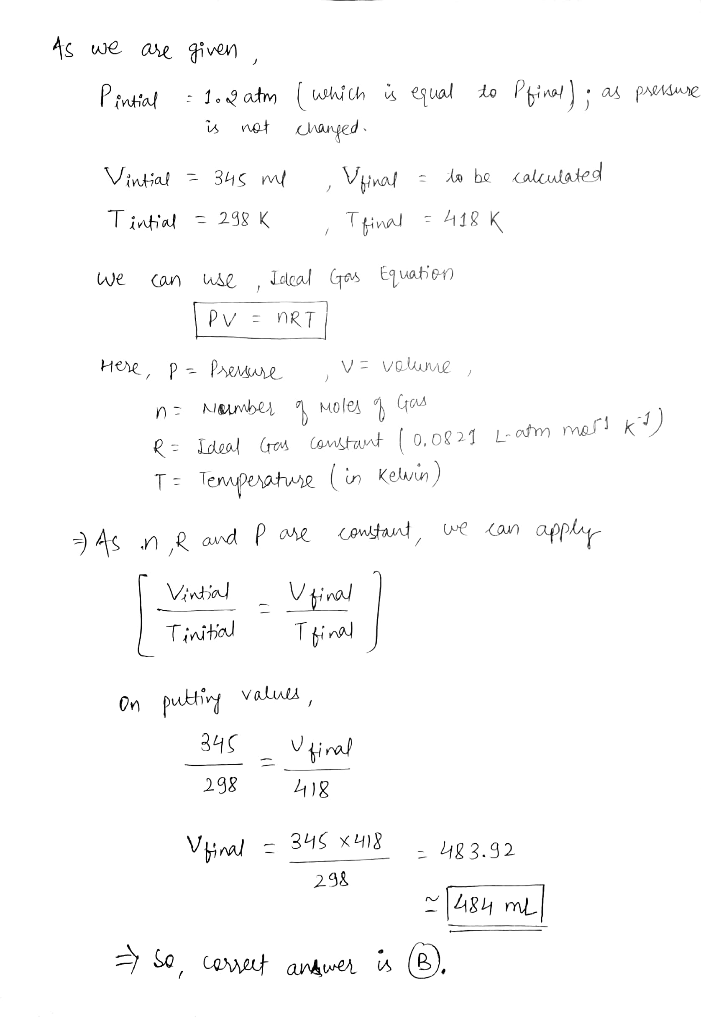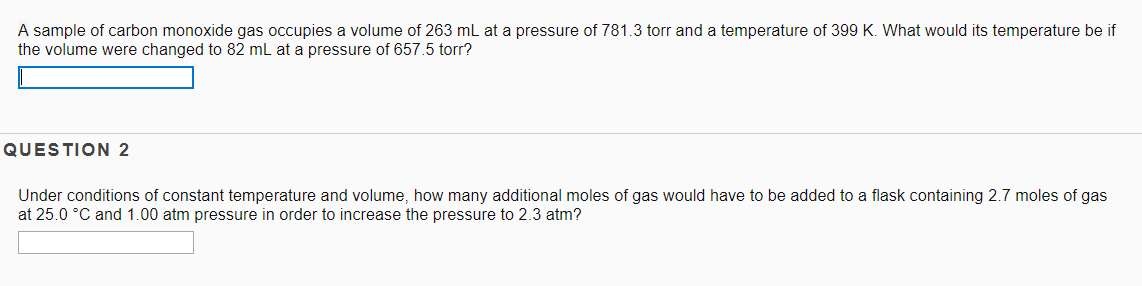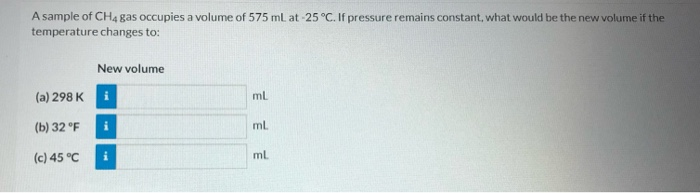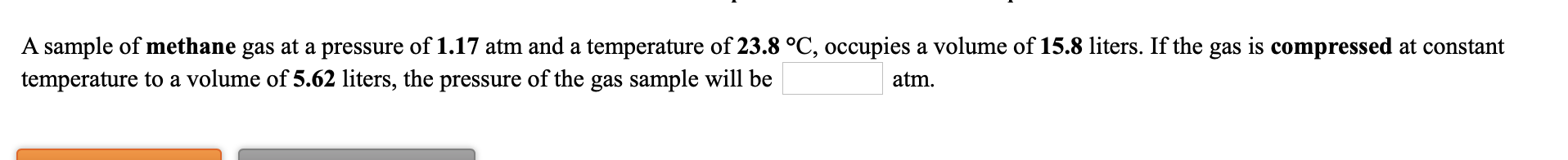Question

# A sample of ethane occupies 345 mL at 298 K and 1.2 atm. If the pressure...

A sample of ethane occupies 345 mL at 298 K and 1.2 atm. If the pressure is not changed, what is the volume of this sample at 418 K?

A) 246 mL

B) 484 mL

C) 414 mL#### Earn Coins

Coins can be redeemed for fabulous gifts.

Similar Homework Help Questions
• ### A sample of air occupies 3.8 L when the pressure is 1.2 atm. a volume does...

A sample of air occupies 3.8 L when the pressure is 1.2 atm. a volume does it occupy at 6.6 atm? (b) What pressure is required compress it to 0.075 L? (The temperature is kept constant.) to

• ### A sample of carbon monoxide gas occupies a volume of 263 mL at a pressure of...A sample of carbon monoxide gas occupies a volume of 263 mL at a pressure of 781.3 torr and a temperature of 399 K. What would its temperature be if the volume were changed to 82 mL at a pressure of 657.5 torr? QUESTION 2 Under conditions of constant temperature and volume, how many additional moles of gas would have to be added to a flask containing 2.7 moles of gas at 25.0 °C and 1.00 atm pressure in order...

• ### A 0.875 g sample of an unknown compound occupies 245 mL at 298 K and 1.22 atm. What is the molar mass of the unknown com...

A 0.875 g sample of an unknown compound occupies 245 mL at 298 K and 1.22 atm. What is the molar mass of the unknown compound?

• ### A 0.415 g sample of an unknown compound occupies 235 mL at 298 K and 1.9...

A 0.415 g sample of an unknown compound occupies 235 mL at 298 K and 1.9 atm. What is the molar mass of the unknown compound?

• ### A sample of CH4 gas occupies a volume of 575 ml at -25°C. If pressure remains...A sample of CH4 gas occupies a volume of 575 ml at -25°C. If pressure remains constant, what would be the new volume if the temperature changes to: New volume (a) 298 K ml (b) 32 °F i ml (c) 45 °C ml

• ### 1.  If a gas occupies 2 L at 3 atm, how many liters will it occupy at...

1.  If a gas occupies 2 L at 3 atm, how many liters will it occupy at 6 atm if the temperature is not changed? a)1L b)2L c)3L d)4L 2. IF A GAS OCCUPIES 2 L AT 273 °C, WHAT IS THE VOLUME AT 0 °C IF THE PRESSURE DOES NOT CHANGE? a)0.5 L b)1 L c)2 L d)4 L 3. If a gas occupies 5 L at 298 K, what temperature is needed to reduce the volume to 2 L...

• ### A sample of oxygen has a volume of 450 mL when its pressure is 1.2 atm....

A sample of oxygen has a volume of 450 mL when its pressure is 1.2 atm. What will the volume of the gas be at a pressure of 1.15 atm if the temperature remains constant?

• ### a sample of gas at 0.75 atm occupies a volume of 521 mL. if the temperature...

a sample of gas at 0.75 atm occupies a volume of 521 mL. if the temperature remains constant, what will be the new pressure if the volume increases to 776 ml ?

• ### 2) Consider a sample of gas that occupies 23.1 L at 1.13 atm. What volume will...

2) Consider a sample of gas that occupies 23.1 L at 1.13 atm. What volume will the same sample occupy if the pressure is increased to 3.05 atm while the temperature is held constant? 3) A fixed sample of gas occupies 25.9 mL at 211.3 K. What volume will the gas occupy at 336.6 K? 4) A fixed sample of gas exerts a pressure of 580 Torr at 284 K. What will the pressure be (in atm) if the temperature...

• ### A sample of methane gas at a pressure of 1.17 atm and a temperature of 23.8...A sample of methane gas at a pressure of 1.17 atm and a temperature of 23.8 °C, occupies a volume of 15.8 liters. If the gas is compressed at constant temperature to a volume of 5.62 liters, the pressure of the gas sample will be atm. A sample of oxygen gas at a pressure of 0.976 atm and a temperature of 22.7 °C, occupies a volume of 786 mL. If the gas is allowed to expand at constant temperature until...